# Foundations

These are some of the known facts about game-theoretic upper probability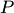:

• It is an outer measure [obvious].
• It is a Choquet capacity, at least in the case of a finite outcome space and one-step ahead forecasts [Vovk 2009].
• In general, it is not strongly additive, i.e., it is not guaranteed to satisfy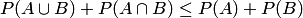. (Therefore, the situation is similar to that in the theory of imprecise probabilities: cf. Walley (2000), page 128.) This is a simple example in the prequential framework (sequential probability forecasting of binary outcomes) with horizon 2 (i.e., the forecaster issues 2 forecasts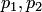for 2 consecutive outcomes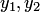):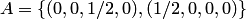and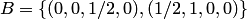(the elements of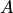and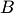are represented in the form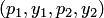). In this case we have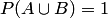and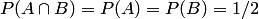. (For the standard prequential framework with infinite horizon just add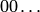at the end of each element ofand.)

Bibliography

• Vladimir Vovk. Prequential probability: game-theoretic = measure-theoretic. The Game-Theoretic Probability and Finance Project,Working Paper 27, January 2009.
• Peter Walley. Towards a unified theory of imprecise probability. International Journal of Approximate Reasoning 24 (2000) 125 - 148.